• 傅里叶级数指数展开
千次阅读
2021-09-20 19:00:06

# 傅里叶级数展开

## 傅里叶级数展开原理

周期函数可以通过一系列的三角函数的线性组合来逼近,傅里叶技术展开可以找到这些三角函数.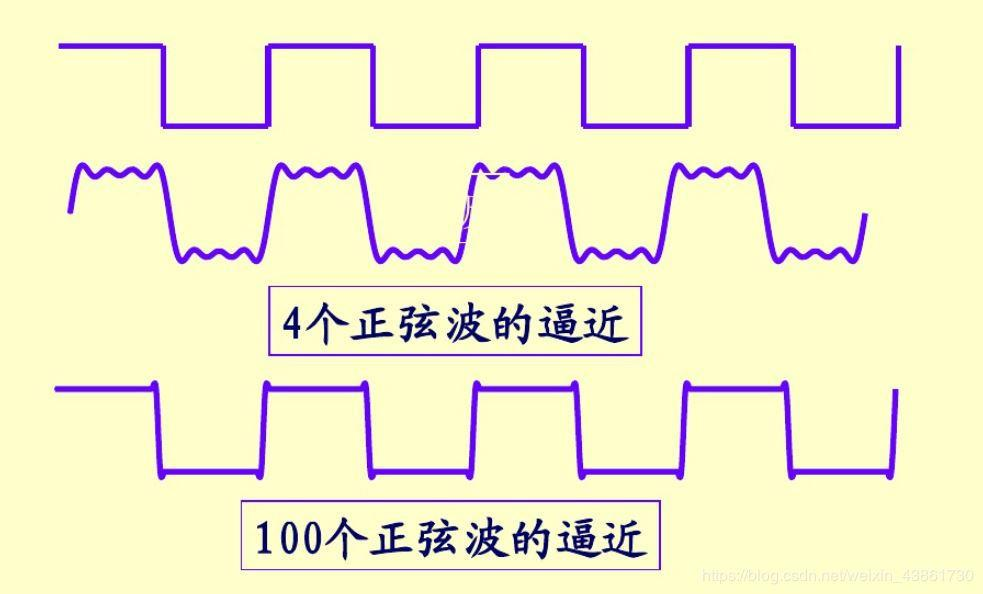首先我们可以通过三角函数的正交性计算出傅里叶级数中的系数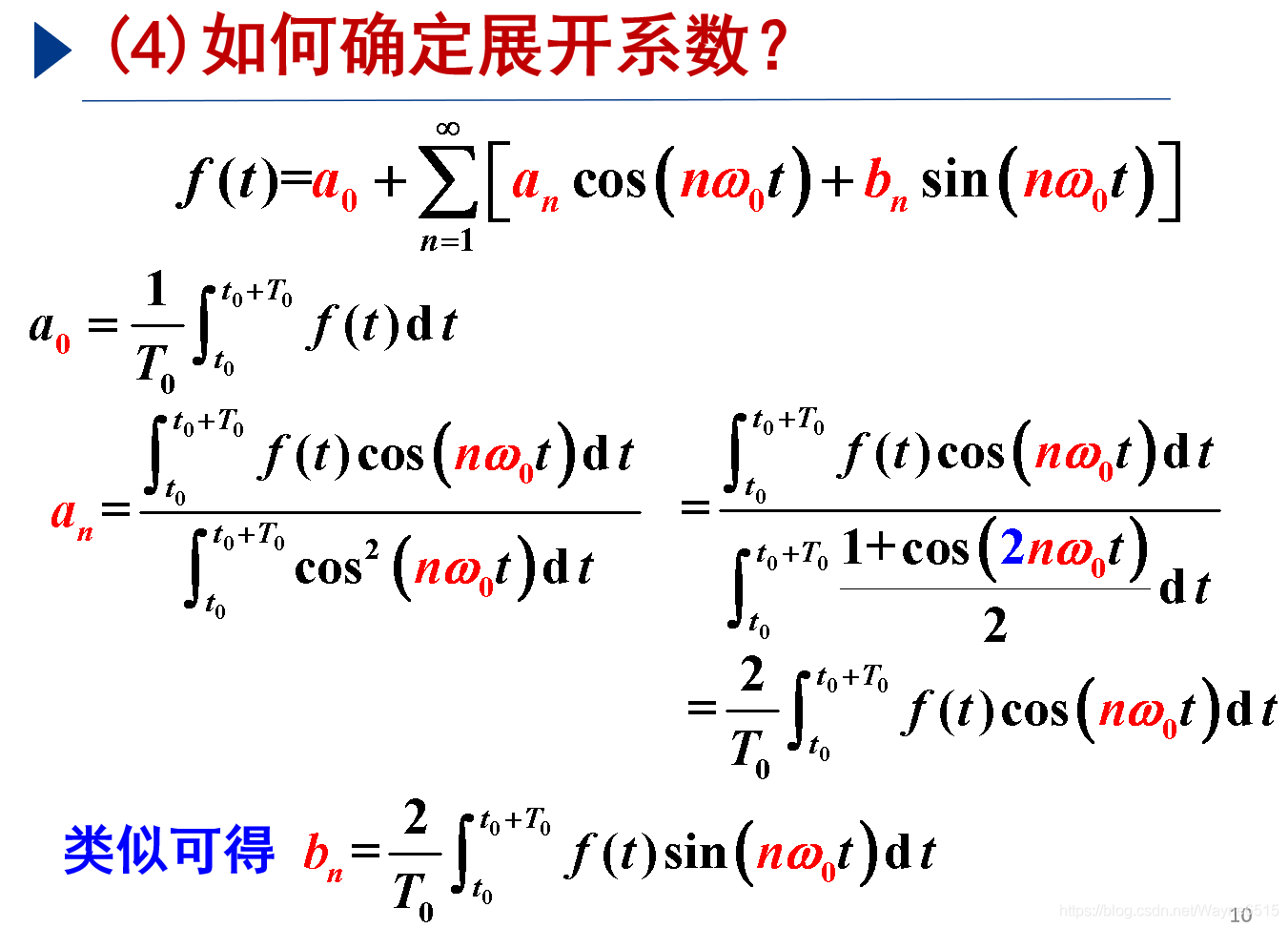## 源码

### 方波


function F=fouriersquare
syms x;       %创建变量x
T=0.00001;
i=100;          %谐波的阶数 数字越大越接近直线
t=0:0.00000001:0.0001; %采样点实际使用可调大
%如果创建-1,+1的方波直接调用square即可
%40是40%占空比
f=max(0.7055*square(pi*2*t/0.00001,40),0);
%创建方波最大值是0.7055，最小值是0 除周期
%square(t,duty_cycle)产生一个周期为2 π,幅值为±1的周期性方波, duty_cycle占空比
%square输入的数小于pi，就是1，大于pi小于2*pi就是-1。
%max取正数部分
plot(t,f);
grid on;
hold on;
axis([0 5*10^(-5) -0.5 1.5]);
A0=0.7055*2/5;%可根据函数直接算出
F=0;
Fx=0;
for n=1:i
As=int(0.7055*2*cos(2*pi*n*x/T)/T,x,0,2*T/5);%傅里叶系数an M = int ( fn, x, xmin, xmax )一重积分
Bs=int(0.7055*2*sin(2*pi*n*x/T)/T,x,0,2*T/5);%傅里叶系数bn
F=F+As*cos(2*pi*n*t/T)+Bs*sin(2*pi*n*t/T);%求傅里叶级数展开
Fx=Fx+As*cos(2*pi*n*x/T)+Bs*sin(2*pi*n*x/T);
end
F=F+A0;
Fx=Fx+A0;
Fx
%figure(2)
plot(t,F)



### 方波＋三角波

function F=fourier_square(amplitude,duty_cycle,T,slope)
syms x amp;
%求傅里叶展开
%amplitude 幅值
%duty_cycle 占空比
%T 周期
%slope 斜率
%amplitude=0.7055;
%duty_cycle=0.4;
%T=0.00001;
%slope=62125;
i=20;              %谐波的阶数 数字越大越接近直线
t=0:T/1000:T*10;    %采样点范围,调大更精确
deviant=(0.5-duty_cycle)*2;
f=max(slope*T/2*((sawtooth((2*t/T+1.0+deviant)*pi)-deviant)),0)+max(amplitude*square(pi*2*t/T,duty_cycle*100),0);
plot(t,f);
grid on;
hold on;
axis([0 T*5 -0.5*amplitude 1.5*amplitude]);
A0=amplitude*duty_cycle+1/2*slope*duty_cycle^2*T;
F=0;
Fx=0;
for n=1:i
As=int(2*(amplitude+slope*x)*cos(2*pi*n*x/T)/T,x,0,T*duty_cycle);%傅里叶系数an M = int ( fn, x, xmin, xmax )一重积分
Bs=int(2*(amplitude+slope*x)*sin(2*pi*n*x/T)/T,x,0,T*duty_cycle);%傅里叶系数bn
end
F=F+As*cos(2*pi*n*t/T)+Bs*sin(2*pi*n*t/T);              %求傅里叶级数展开
Fx=Fx+As*cos(2*pi*n*x/T)+Bs*sin(2*pi*n*x/T);
end
F=F+A0;
Fx=Fx+A0;
Fx
plot(t,F)

简单说明一下函数,本逻辑将电流分成三角波和方波的周期函数叠加,分别做傅里叶分 解。函数需要输入周期函数的周期、方波的幅值、周期函数的占空比、以及三角波的斜率。 首先作出方波和三角波函数叠加后的图像，而后将函数进行傅里叶分解，同时输出傅里叶函 数直流、基波、二次谐波、三次谐波的幅值和角度用于 Ansys Maxwell 仿真时候加激励源， 最后画出傅里叶展开设定次数内函数叠加的图片用于原函数对比傅里叶展开的收敛程度。函 数内有两个可调值 i 和 t，i 为傅里叶分解的谐波的阶数，数字越大越接近直线；t 为采样 点范围,数据越小越精确，但调大会让仿真时间较长。
由于 matalb 只有几种周期函数,因此实现目标电流函数需要通过位移、函数取正、更改 周期来实现。


## matalb 傅里叶级数展开结果图片

结果展示： 收敛 i=20 结果仍有较多毛刺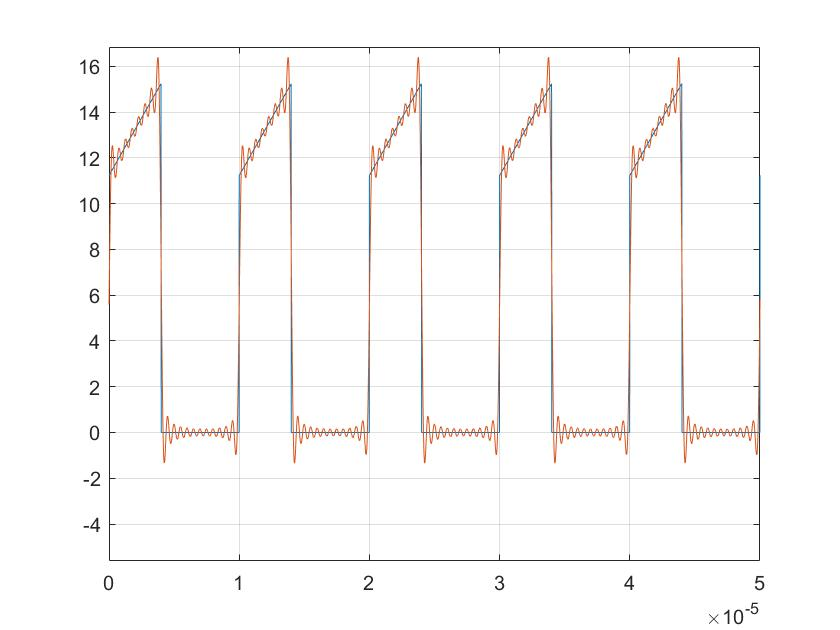收敛 i=50 时候较为接近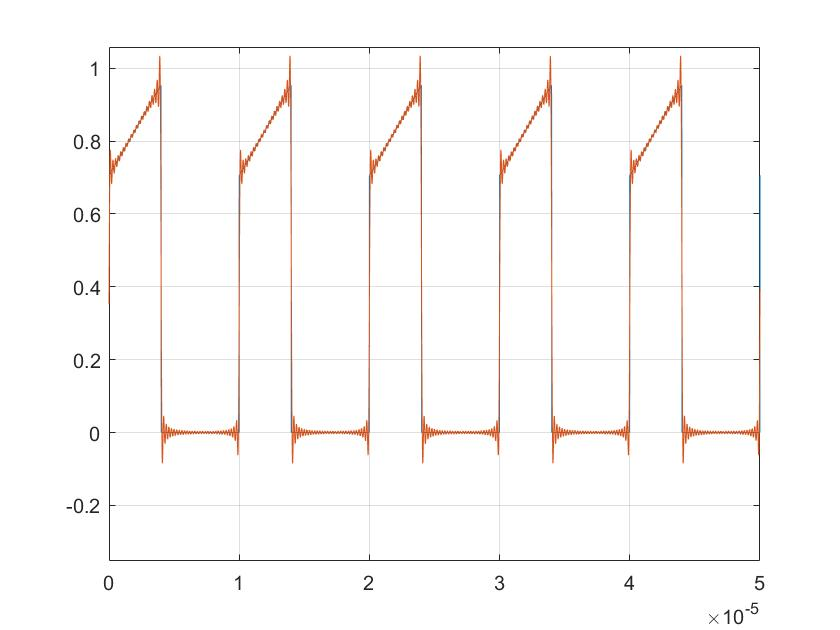i 继续增大仿真时间指数增加，因此没有继续，理论上 i 无限大时会与原曲线重合，从而验 证傅里叶分解正确性

补充:实际matlab好像有自己傅里叶分解函数fft(),可以通过help查看用法,自己编写函数会掉一些精度matlab
更多相关内容
• 三角形式的傅里叶级数，含义比较明确，但运算常感不便，因而经常采用指数形式的傅里叶级数。 这里利用到了欧拉公式 cosx=ejx+e−jx2cosx=\frac{e^{jx}+e^{-jx}}{2}cosx=2ejx+e−jx​

三角形式的傅里叶级数，含义比较明确，但运算常感不便，因而经常采用指数形式的傅里叶级数。
这里利用到了欧拉公式
c o s x = e j x + e − j x 2 cosx=\frac{e^{jx}+e^{-jx}}{2}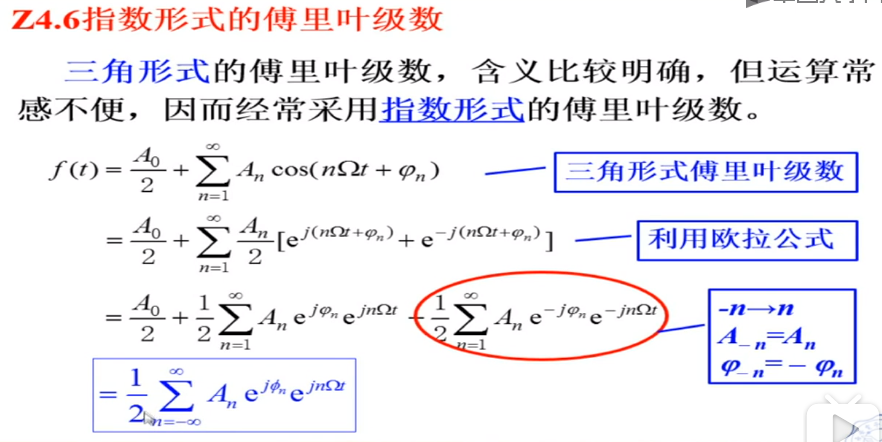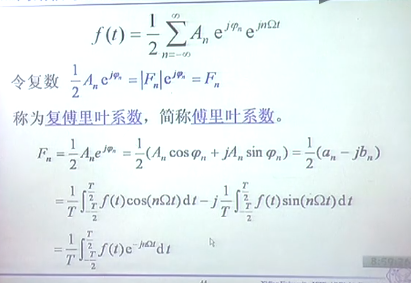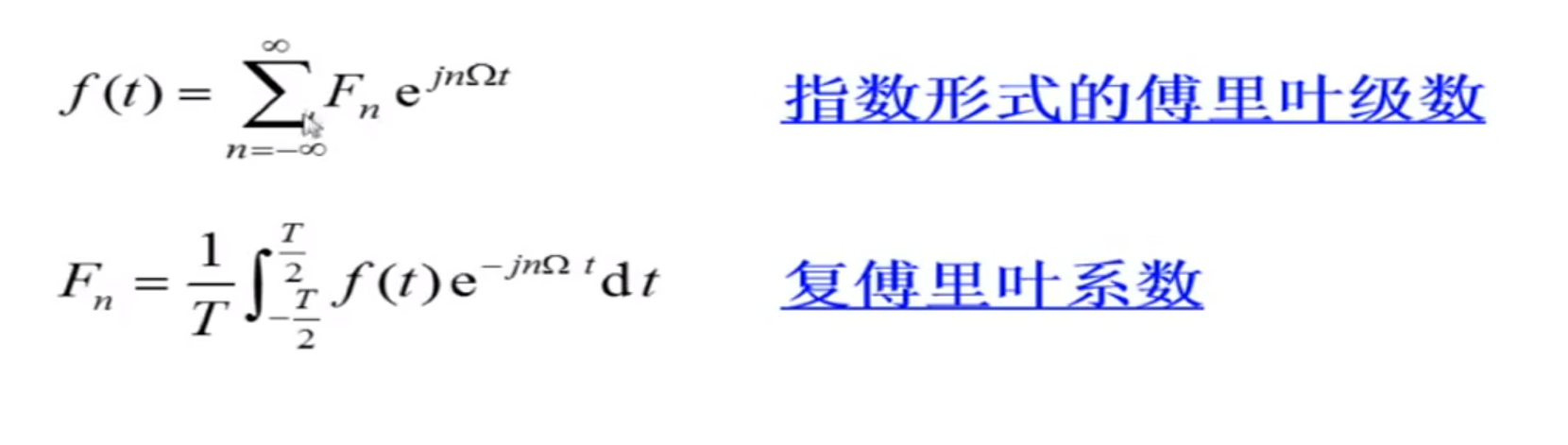这个式子表明：任意周期信号可以分解为许多不同频率的虚指数信号之和。 F n F_n 是频率为 n Ω n\Omega 的分量的系数， F 0 = A 0 / 2 F_0=A_0/2 为直流分量。

展开全文• 引言近来，在开展课题时遇到了需要将梯形波进行傅里叶级数展开的问题，查询了一些资料(惭愧，一开始就没想着自己动手积分)，然后没有找到自己想要的结果(其实有相近的，只不过不是任意周期的，当时没有转变过来)，...

引言

近来，在开展课题时遇到了需要将梯形波进行傅里叶级数展开的问题，查询了一些资料(惭愧，一开始就没想着自己动手积分)，然后没有找到自己想要的结果(其实有相近的，只不过不是任意周期的，当时没有转变过来)，最后还是动手算出来了，在这里做一个小小的记录，算是回顾以前的知识吧，捂脸。

由于像三角波，矩形波，梯形波这种波形不连续，因此在仿真软件中很容易出现计算不收敛的情况。所以，在这种情况下，利用一系列谐波叠加的形式来等价于原来的波形，可以很好的优化模型。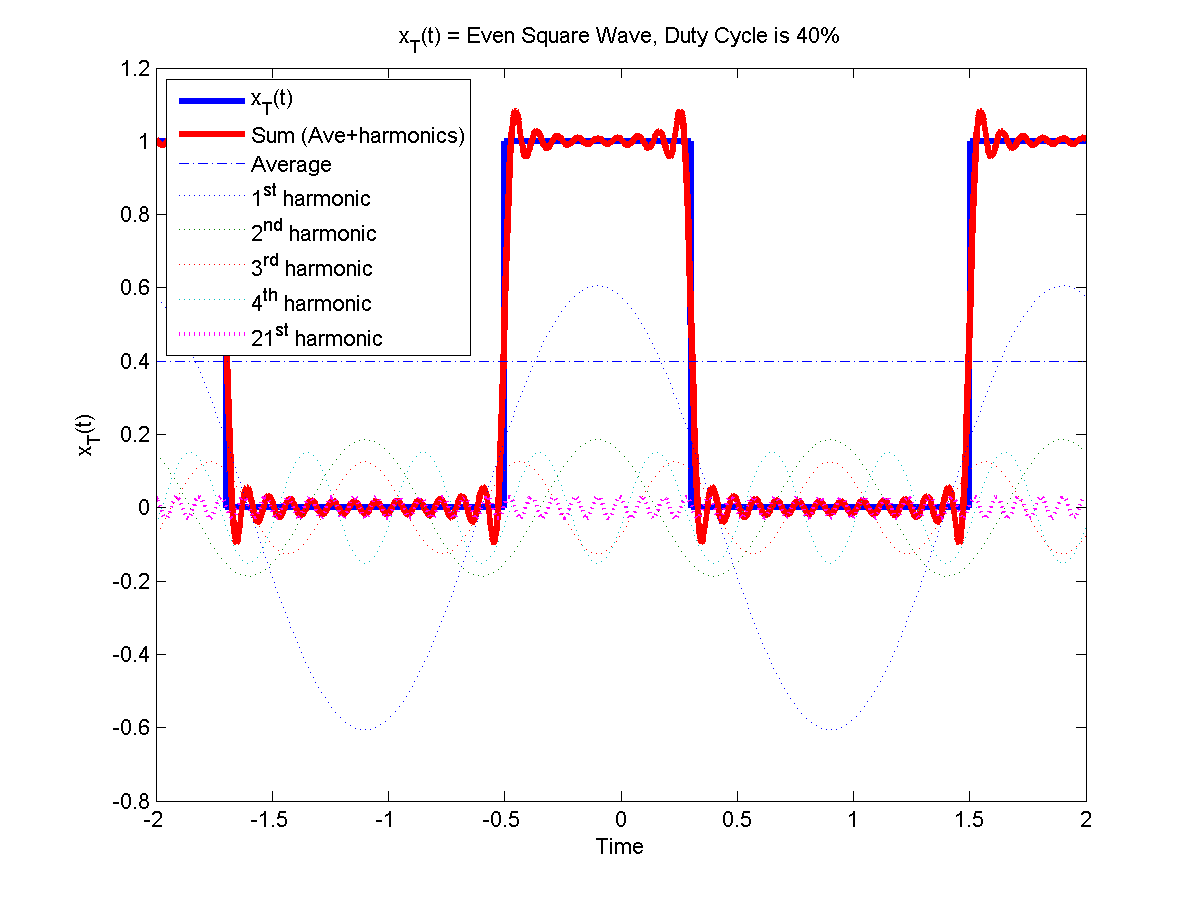预备知识

公式

给定一个周期为 $$T$$ 的函数 $$x(t)$$ ，那么它可以表示为无穷级数：

$f(x) \sim \frac{a_0}{2} + \sum_{n=1}^{\infty} \left [a_n \cos \left (\frac{2 {\pi} nx}{T} \right ) + b_n \sin \left (\frac{2 {\pi} nx}{T} \right ) \right ] = \sum_{n=-\infty}^{\infty} c_n e^{i \frac{2 {\pi} nx}{T} }$

其中傅里叶系数为：

\left \{ \begin{aligned} a_n = &\frac{2}{T} \int_{t_0}^{t_0+T} f(t) \cdot \cos \left (\frac{2 {\pi} nt}{T} \right )dt \qquad &n=0, 1, 2, \cdots \\[2ex] b_n = &\frac{2}{T} \int_{t_0}^{t_0+T} f(t) \cdot \sin \left (\frac{2 {\pi} nt}{T} \right )dt &n=1, 2,3, \cdots \\[2ex] c_n = &\frac{1}{T} \int_{t_0}^{t_0+T} f(t) \cdot e^{-i \frac{2 {\pi} nt}{T} }dt &n=0, \pm 1, \pm 2, \cdots \end{aligned} \right.

性质

收敛性

在闭区间上满足狄利克雷条件的函数表示成的傅里叶级数都收敛。狄利克雷条件如下：

在定义区间上，$$x(t)$$需绝对可积；

在任一有限区间中，$$x(t)$$只能取有限个极值点；

在任何有限区间上，$$x(t)$$只能有有限个第一类间断点。

满足上述条件的$$x(t)$$傅里叶级数都收敛，且：

当$$t$$是$$x(t)$$的连续点时，级数收敛于$$x(t)$$

当$$t$$是$$x(t)$$的间断点时，级数收敛于$$\frac{1}{2} \left [x(t^-)+x(t^+) \right ]$$

正交性

所谓的两个不同向量正交是指它们的内积为0，这也就意味着这两个向量之间没有任何相关性，例如，在三维欧式空间中，互相垂直的向量之间是正交的。三角函数族的正交性用公式表示出来就是：

\left\{ \begin{aligned} &\int_0^{2 \pi} \cos(mx) \cdot \cos(nx) dx =0 \qquad (m \ne n) \\[2ex] &\int_0^{2 \pi} \sin(nx) \cdot \sin(nx) dx = \pi \\[2ex] &\int_0^{2 \pi} \cos(nx) \cdot \cos(nx) dx = \pi \end{aligned} \right.

奇偶性

奇函数$$f_o(x)$$可以表示为正弦级数，而偶函数$$f_e(x)$$则可以表示成余弦级数：

\begin{aligned} f_o(x) &\sim \sum_{n=1}^{\infty} b_n \sin \left (\frac{2 {\pi} nx}{T} \right ) \\ f_e(x) &\sim \frac{a_0}{2} + \sum_{n=1}^{\infty} a_n \cos \left (\frac{2 {\pi} nx}{T} \right )\end{aligned}

几种常见波形的傅里叶级数展开式

梯形波(奇函数)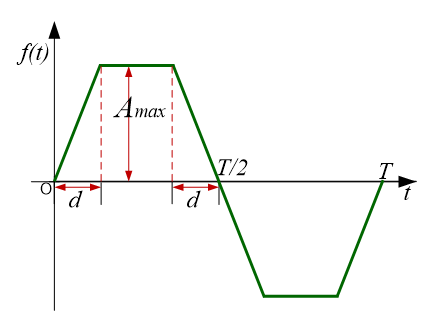如上图所示，该梯形波是一个周期为T的奇函数，幅值为$$A_{max}$$，上升沿时间为$$d$$，在区间$$\left [0, \frac{T}{2} \right ]$$的函数表达式为：

$f(t) = \begin{cases} \frac {A_{max} }{d} t, \qquad \qquad & 0 \le t \le d \\[2ex] A_{max}, & d \le t \le \frac{T}{2} - d \\[2ex] \frac {A_{max} }{d} \left (\frac{T}{2} - t \right ), & \frac{T}{2} - d \le t \le \frac{T}{2} \end{cases}$

由奇偶性可知，该波形在区间$$\left [-\frac{T}{2}, \frac{T}{2} \right ]$$的傅里叶级数展开式为：

$f(t) \sim \sum_{n=1}^{\infty} b_n \sin \left (\frac{2 {\pi} nt}{T} \right )$

其中傅里叶系数为：

$b_n = \frac{2}{T} \int_{-\frac{T}{2} }^{\frac{T}{2} } f(t) \cdot \sin \left (\frac{2 {\pi} nt}{T} \right )dt \quad \quad n=1, 2,3, \cdots$

将$$f(t)$$函数代入傅里叶系数表达式中，可得：

\begin{aligned} b_n &= \frac{2}{T} \int_{-\frac{T}{2} }^{\frac{T}{2} } f(t) \cdot \sin \left (\frac{2 {\pi} nt}{T} \right )dt = \frac{4}{T} \int_{0}^{\frac{T}{2} } f(t) \cdot \sin \left (\frac{2 {\pi} nt}{T} \right )dt \\[2ex] &= \frac{4}{T} \left [ \int_0^d \frac{A_{max} }{d} t \cdot \sin \left (\frac{2 {\pi} nt}{T} \right )dt + \int_d^{\frac{T}{2} - d} A_{max} \cdot \sin \left (\frac{2 {\pi} nt}{T} \right )dt + \int_{\frac{T}{2} - d}^{\frac{T}{2} } \frac{A_{max} }{d} \left (\frac{T}{2}-t \right ) \cdot \sin \left (\frac{2 {\pi} nt}{T} \right )dt \right ] \\[2ex] &= \left . \left . \frac{4}{T} \left [ -\frac{A_{max} }{d} {T \over {2 \pi n} } \cdot t \cdot \cos \left ( \frac{2\pi nt}{T} \right ) \right | _0^d + {A_{max} \over d } {T^2 \over 4 \pi^2 n^2} \cdot \sin \left( {2 \pi n t \over T} \right ) \right | _0^d \right ] + {4 \over T} \left [ \left . - {A_{max}T \over 2 \pi n} \cdot \cos \left( {2\pi nt \over T}\right) \right |_d^{ {T \over 2}-d} \right ] + {} \\[2ex] &{} + \left. {4 \over T} \left [ -{A_{max} \over d} {T \over 2 \pi n} \cdot \left ( {T \over 2} - t \right ) \cdot \cos \left ( \frac{2\pi nt}{T} \right ) \right | _{ {T \over 2} - d}^{T \over 2} - \left . {A_{max} \over d}{T^2 \over 4 \pi^2 n^2} \cdot \sin \left( {2 \pi n t \over T} \right ) \right | _{ {T \over 2} - d}^{T \over 2} \right ] \\[2ex] &= {4 \over T} \left \{ {A_{max} T^2 \over 4d \pi ^2 n^2} \left [ \sin \left( {2 \pi n \over T} \cdot d \right) + \sin \left( {2 \pi n \over T} \cdot \left( {T \over 2} - d \right ) \right) \right ] \right \} ={A_{max} T \over d \pi ^2 n^2} \left[ \sin\left( {2 \pi n d \over T} \right) + \sin \left( n\pi - {2 \pi n d \over T} \right) \right] \end{aligned}

由 $\sin \left( n\pi - {2 \pi n d \over T} \right) = \begin{cases} \sin\left( {2 \pi n d \over T} \right) \qquad \qquad &n=2N-1 \\[2ex] -\sin\left( {2 \pi n d \over T} \right) &n=2N \end{cases}$ 可得：

$b_n = \begin{cases} {2 A_{max} T \over d \pi ^2 n^2} \left[ \sin\left( {2 \pi n d \over T} \right) \right ] \qquad \qquad &n=2N-1 \\[2ex] 0 &n=2N \end{cases}$

综上所述，可以得到该梯形波在区间$$\left[ -{T \over 2}, {T \over 2} \right ]$$的傅里叶级数展开式为：

$f(t) \sim {4 A_{max} \over \pi \omega d} \sum _{n=1}^{\infty} {\sin ( { (2n-1) \omega d }) \over (2n-1)^2} \cdot \sin((2n-1) \omega t) \qquad n=1,2,3,\cdots$ 其中：$$\omega = {2 \pi \over T}$$

脉冲波(偶函数)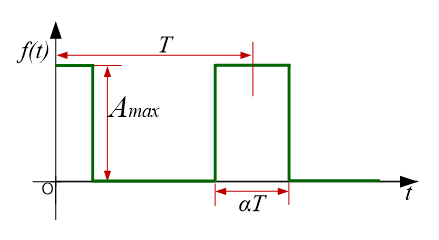如上图所示，该脉冲波是一个周期为T的偶函数，幅值为$$A_{max}$$，脉冲宽度为$$\alpha T$$，在区间$$\left[ -{T \over 2}, {T \over 2} \right ]$$的函数表达式为：

$f(t)= \begin{cases} A_{max}, \quad &|t| \le { {\alpha T} \over 2} \\[2ex] 0, &|t| \gt { { {\alpha T} } \over 2} \end{cases}, \qquad - {T \over 2} \le t \le {T \over 2}$

由奇偶性可知，该波形在区间$$\left[ -{T \over 2}, {T \over 2} \right ]$$的傅里叶级数展开式为：

$f(t) \sim \frac{a_0}{2} + \sum_{n=1}^{\infty} a_n \cos \left (\frac{2 {\pi} n t}{T} \right )$

其中傅里叶系数为：

a_n =\left\{\begin{aligned} &{2 \over T} \int_{-{T \over 2} }^{T \over 2} f(t) dt \qquad \qquad \qquad \qquad & n=0 \\[2ex] &\frac{2}{T} \int_{-\frac{T}{2} }^{\frac{T}{2} } f(t) \cdot \cos \left (\frac{2 {\pi} nt}{T} \right )dt & n=1, 2,3, \cdots \end{aligned}\right.

将$$f(t)$$函数代入傅里叶系数表达式中，可得：

\begin{aligned} a_0 &= {2 \alpha A_{max} } \\[2ex] a_n &={2 \over T} \int _{-{\alpha T \over 2} }^{\alpha T \over 2} A_{max} \cos \left (\frac{2 {\pi} nt}{T} \right )dt = {4 \over T} \int _{0}^{\alpha T \over 2} A_{max} \cos \left (\frac{2 {\pi} nt}{T} \right )dt\\[2ex] &= \left . {4 \over T} {A_{max} T \over 2 \pi n } \sin \left( {2 \pi n t \over T} \right) \right|_0^{\alpha T \over 2} ={2A_{max} \over n \pi} \sin (\alpha n \pi) \qquad \qquad \qquad \qquad n=1,2,3,\cdots \end{aligned}

因此，可以得到该梯形波在区间$$\left [ -{T \over 2}, {T \over 2} \right ]$$的傅里叶级数展开式为：

$f(t) \sim \alpha A_{max} + {2A_{max} \over \pi} \sum _{n=1}^{\infty}{\sin (\alpha n \pi) \over n} \cos(n\omega t) \qquad n=1,2,3, \cdots$

其中：$$\omega = {2 \pi \over T}$$

方波(奇函数)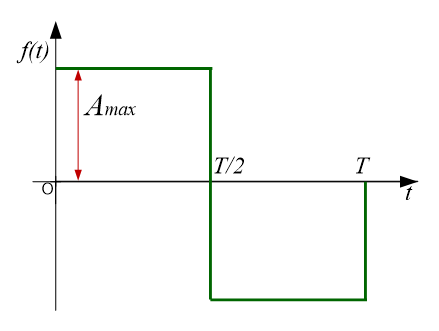同理，该方波在区间$$\left [ -{T \over 2}, {T \over 2} \right ]$$的傅里叶级数展开式为：

$f(t) \sim {4A_{max} \over \pi} \sum_{n=1}^{\infty}{\sin((2n-1)\omega t) \over 2n-1} \qquad \qquad n=1,2,3,\cdots$

其中：$$\omega = {2 \pi \over T}$$

三角波(奇函数)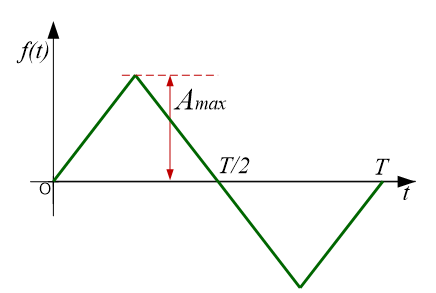同理，该三角波在区间$$\left [ -{T \over 2}, {T \over 2} \right ]$$的傅里叶级数展开式为：

$f(t) \sim {8A_{max} \over \pi^2}\sum_{n=1}^{\infty}{(-1)^{n-1}\sin((2n-1)\omega t) \over (2n-1)^2} \qquad n=1,2,3,\cdots$

锯齿波(非奇非偶函数)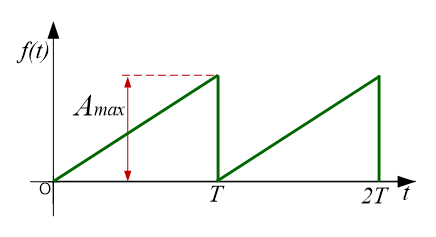该锯齿波如上图所示，在区间$$[0, T]$$的函数表达式为：

$f(t)={A_{max} \over T}t \qquad \qquad 0 \le t \le T$

由于该函数为非奇非偶函数，因此，该波形在区间$$[0, T]$$的傅里叶级数展开式为：

$f(x) \sim \frac{a_0}{2} + \sum_{n=1}^{\infty} \left [a_n \cos \left (\frac{2 {\pi} nx}{T} \right ) + b_n \sin \left (\frac{2 {\pi} nx}{T} \right ) \right ]$

其中傅里叶系数为：

\begin{aligned} a_n &= \frac{2}{T} \int_{0}^{T} f(t) \cdot \cos \left (\frac{2 {\pi} nt}{T} \right )dt \qquad \qquad & n=0, 1, 2, \cdots \\[2ex] b_n &= \frac{2}{T} \int_{0}^{T} f(t) \cdot \sin \left (\frac{2 {\pi} nt}{T} \right )dt & n=1, 2,3, \cdots \end{aligned}

将$$f(t)$$函数代入傅里叶系数表达式中，可得：

\begin{aligned} a_0 &=A_{max} \\[2ex] a_n &= {2 \over T} \int_0^T{A_{max} \over T}t \cdot \cos \left( {2 \pi nt\over T} \right) dt \\[2ex] & = {2 \over T} {A_{max} \over T}{T \over 2 \pi n} \left . \left . \left[ t\sin \left({2\pi nt \over T}\right) \right| _0^T + {T \over 2 \pi n} \cos \left({2\pi nt \over T}\right) \right| _0^T \right] =0 \qquad &n=1,2,3,\cdots\\[2ex] b_n &= {2 \over T} \int_0^T{A_{max} \over T}t \cdot \sin \left( {2 \pi nt\over T} \right) dt \\[2ex] &= -{2 \over T} {A_{max} \over T}{T \over 2 \pi n} \left . \left . \left[ t\cos \left({2\pi nt \over T}\right) \right| _0^T - {T \over 2 \pi n}\sin \left({2\pi nt \over T}\right) \right| _0^T \right] =-{A_{max} \over n\pi} &n=1,2,3,\cdots \end{aligned}

因此，可以得到该锯齿波在区间$$[0,T]$$的傅里叶级数展开式为：

$f(t) \sim {A_{max} \over 2}-{A_{max} \over \pi} \sum_{n=1}^{\infty} {\sin(n \omega t) \over n} \qquad \qquad n=1,2,3,\cdots$

结语

这里仅仅列出了极小部分的波形的傅里叶级数展开式，对于其它波形，类似代入计算即可，给出公式之后，更多的是考验数学积分计算了。

参考文献

 维基百科编者. 傅里叶级数

 百度百科编者. 傅里叶级数

展开全文• 傅里叶提出的两个重要的观点： 1.周期信号都可以表示为谐波关系的正弦信号的加权和（傅里叶级数） 2.非周期信号都可用正弦信号的加权积分表示（傅里叶变换） 参考上一篇博客 ...得指数形式的傅里叶级数 由于

傅里叶提出的两个重要的观点：
1.周期信号都可以表示为谐波关系的正弦信号的加权和（傅里叶级数）
2.非周期信号都可用正弦信号的加权积分表示（傅里叶变换）

参考上一篇博客
https://blog.csdn.net/Chevy_cxw/article/details/110948262
对三角函数形式的傅里叶级数使用欧拉公式
欧拉公式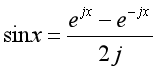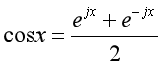三角函数形式的傅里叶级数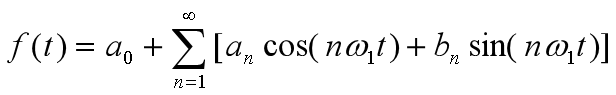将欧拉公式代入三角函数形式的傅里叶级数有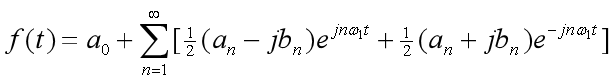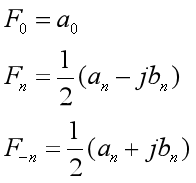an 是关于n的偶函数， bn是关于n的奇函数
代入化简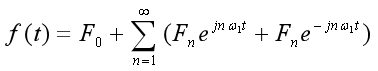得指数形式的傅里叶级数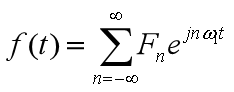由于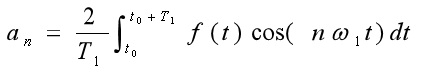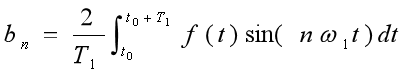在Fn中套入欧拉公式有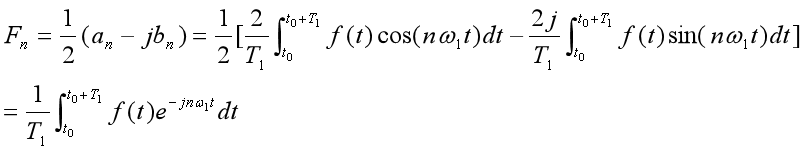得到变换对
综合公式分析公式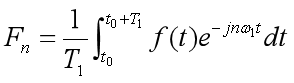周期信号可分解为负无穷到无穷大区间上的指数信号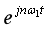的线性组合。

Fn为频谱函数，是频率的函数，它反映了组成信号各正弦谐波的幅度和相位随频率变化的规律。如果给出Fn，则f(t)唯一确定。

参考书籍：
信号与系统（第二版）中文版，奥本海姆
信号与系统（第二版）上册，郑君里

展开全文• 这里的程序绘制了用户给定 'Ck' 的周期信号图。... 我们在这里使用术语信号和函数（两者在这里可以互换） 众所周知，我们可以用复指数函数来描述任何周期函数。 复系数的系数用“Ck”表示。 Ck 是'K' 的函数。matlab
• 其实有两种方法，第一种方法较为简单： 方法一：利用复指数函数的展开式 已知： (1)-(2)可得： 积分计算方法 所以：
• 周期锯齿波的三角函数形式的傅里叶级数展开式，共享学习。百度已有，只是这个不用下载券！不用下载券！不用下载券！
• 周期函数的傅里叶级数展开周期函数 周期函数 周期函数表达式为： f(x) = f(x + kT) (k = 1,2,3…) 如果该周期函数满足狄利赫里条件，那么该周期可以展开傅里叶级数： f(t)=a02+∑n=1∞(a0cos⁡(nω1t)+bnsin...
• 名字比较拗口，实际上是：对于一个周期函数，从 三角形式傅里叶级数 引申到 指数形式的傅里叶级数，为什么引申到指数形式，因为加入指数形式后，运算更加简洁，同时引入了方向的概念，因此也有了负频率。 下面是详细...傅里叶变换 傅里叶级数
• 函数 e^(-x) 在区间 (0,2pi) 上的傅立叶级数 (FS)。 这个程序类似于Matlab Demo of Square wave from Sine wave。 它绘制了 e^(-x) 和前 40 项 FS 之间的误差。 由于近似值（忽略 41 到 inf 项）而出现此错误matlab
• ## 方波信号傅里叶级数展开

万次阅读 多人点赞 2020-04-07 17:30:06
周期信号可以进行傅里叶级数展开 在研究非周期信号的傅里叶变换之前 首先应掌握傅里叶级数的三种表述形式： 三角函数形式 谐波形式 指数形式 并根据定义式求出傅里叶系数： 以周期性的方波信号为例，掌握傅里叶...matlab
• 将一个周期信号分解为一个直流分量和一系列复指数信号分量之和的过程被称为傅里叶级数展开。 周期信号f(t)f(t)f(t)的傅里叶级数展开式为：f(t)=∑k=−∞∞ckejkw0tf(t)=\sum_{k=-\infin}^{\infin}c_ke^{jkw_0t}f(t)=...
• 我讨厌傅立叶级数的叫法，这老让我感觉到很深奥，但当我用三角级数时，感觉就大不同了！！ 下面进入正题 正弦波 信号处理中极为极为极为重要的一个函数,三角函数,之所以叫做三角函数,是因为它的计算方式和直角...三角级数
• 文章目录指数形式指数形式推导参考 指数形式 根据欧拉公式： eiθ=cos⁡θ+isin⁡θ e^{i\theta } = \cos \theta +i\sin \theta \\ eiθ=cosθ+isinθ 我们可以推出： sin⁡θ=eiθ−e−iθ2icos⁡θ=eiθ+e−iθ2 \...
• 由三角函数组成的函数项级数，即所谓的三角级数，着重研究如何把函数展开成三角级数。 一、三角级数 三角函数系的正交性 如何深入研究非正弦周期函数呢？我们用函数的幂级数展开式表示与讨论函数，因此我们也可将...
• ## 通俗易懂的傅立叶级数理解

千次阅读 多人点赞 2017-08-17 10:54:57
级数指数）的和。 你知道为什么要展开成幂级数的和吗？请看这里： 因为我们把y展开成泰勒级数 y = 1+x+x^2+x^3+x^4+…的时候我们可以无限细分得到函数在每个点的【【变化】】呀！ 这和你把3234.352拆成3000+...
• ## 傅里叶级数详解

千次阅读 2021-12-28 01:32:25
函数分解为正交函数 1向量的正交分解 2函数的正交分解 周期函数的傅里叶级数 1周期函数三角形式的傅里叶级数 1.1三角形式的傅里叶级数 1.2余弦形...directx winapi svm jetty
• 满足Direchlet条件的周期信号或特定时间区间的信号可以被傅里叶级数在功率上没有误差的表达，常见的傅里叶级数的形式有两种，即： f(t)=a02+∑n=1+∞[ancos(nΩt)+bnsin(nΩt)]an=2t2−t1∫t1t2f(t)cos(nΩt)dtbn=2t...
• ## 傅里叶级数与傅里叶变换

万次阅读 多人点赞 2019-07-19 17:40:05
傅里叶级数：任何周期函数，只要满足一定条件都可以表示为不同频率的正弦和/或余弦之和的形式，该和成为傅里叶级数傅里叶变换：任何非周期函数（但该曲线下的面积是有限的），也可以用正弦和/或余弦乘以加权函数...
• 1. 正余弦信号的复指数表示方法   根据欧拉公式：   将其中的θ替换成ωt，即可得到信号的复指数表达。   可以将该复指数信号看作是一个在单位圆上以角速度ω进行旋转的一个矢量。在旋转的过程中，旋转矢量...傅里叶级数
• 对其进行傅里叶级数展开，计算后可得： 编写的matlab代码如下： close all; clear all; N = 1000; %取展开式的项数为1000项 T = 1; %方波周期为1 fs = 1/T; N_sample = 128; %为了画出波形，...matlab
• 傅里叶分析可分为傅里叶级数（Fourier Serie）和傅里叶变换(Fourier Transformation)，我们从简单的开始谈起。 二、傅里叶级数(Fourier Series)的频谱 还是举个栗子并且有图有真相才好理解。 如果我说我能用...傅里叶变换 傅里叶级数
• 连续时间周期信号的傅里叶三角级数表示1.1 把一个周期函数表示成三角级数1.2 三角函数的正交性1.3 函数展开傅里叶级数2. 连续时间周期信号的傅里叶指数级数表示2.1 周期信号简单介绍2.2 成谐波关系的人工智能
• 　傅里叶级数傅里叶变换、离散傅里叶变换、短时傅里叶变换。。。这些理解和应用都非常难，网上的文章有两个极端：“Esay” Or “Boring”！如果单独看一两篇文章就弄懂傅里叶，那说明你真的是大神了。 　本博文是...机器学习 人工智能 大数据
• 开的这个坑大概就是写写从另一个视角来看快速离散傅里叶变换FFT。oi当中常见的FFT的推导方法...在这个专题下，将会依次讲解傅里叶级数FS，傅里叶变换FT，离散时间傅里叶变换DTFT，离散傅里叶变换DFT。主要是参考wys...
• ## 复指数形式的傅里叶级数

万次阅读 多人点赞 2014-06-24 15:47:18
原文：http://blog.sina.com.cn/s/blog_66585b7f0100hmhq.html  本文详细介绍了傅里叶级数指数变换形式，将指数形式和三角形式相连起来
• (#977) 泰勒级数的基本公式. 这个方程相当于是待解析曲线在求解点附近做了一条切线，并进行迭代法累加(n阶导数)。迭代次数越多，越接近原始曲线。举例用泰勒级数来分解sin(t)，...傅立叶级数的基本公式 　 ...
• 在开始傅里叶变换的推导前，我们先使用欧拉公式把傅里叶级数变成指数形式的，因为指数的积分微分更简单。 关于欧拉公式网上有很多推导视频，如果不想看纯数学推导的同学，可以参考这张图： 这个螺旋前进的轨迹，从......